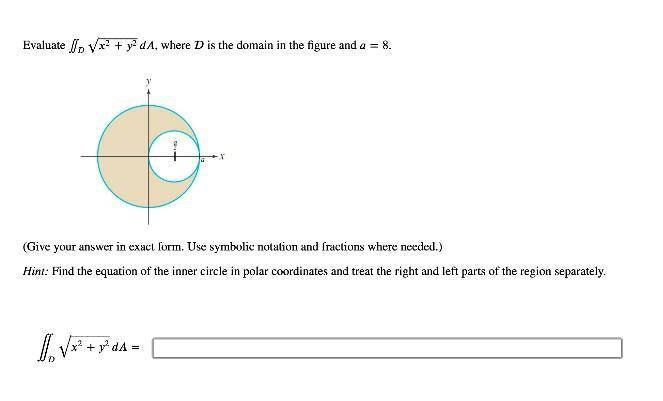Question:

# Evaluate Ip Vx+ + y2dA, where D is the domain in the figure and a = 8. (Give your answer in exact form. Use symbolic notation anEvaluate Ip Vx+ + y2dA, where D is the domain in the figure and a = 8. (Give your answer in exact form. Use symbolic notation and fractions where needed.) Hint: Find the equation of the inner circle in polar coordinates and treat the right and left parts of the region separately. 1. Vx? + gºda = +